(9-8) Select the descriptor (A, B, C, D): that best describes the relationship between the...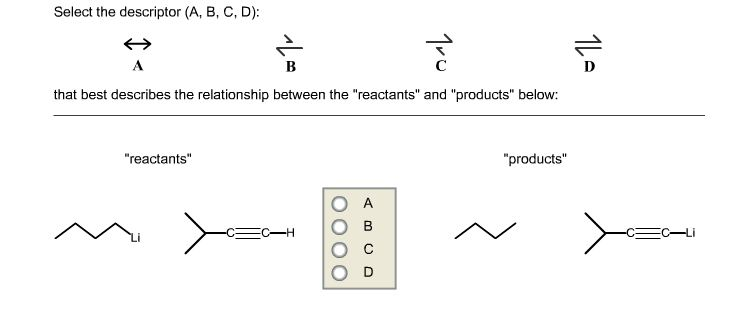Select the descriptor (A, B, C, D): that best describes the relationship between the "reactants" and "products" below:

IOption D

is the descriptor

Option CConcepts and reason

Descriptors: Descriptors are the symbols used to show the transformations from one to another. Descriptors are usually used between reactants and products or between two different structures of the same compound.

Fundamentals

Different types of descriptors are shown below:

A.Double headed arrow: Double headed arrow is shown between the two canonical structures (resonance structures).

B.Equilibrium symbol: Equilibrium symbol, which favors a forward reaction.

C.Equilibrium symbol: Equilibrium symbol which favors a reverse reaction.

D.Equilibrium symbol: Equilibrium symbol, which favors neither forward nor reverse reaction.

Equilibrium nature: Equilibrium shifts towards less acidic substances that are from lower ${\rm{P}}{{\rm{k}}_{\rm{a}}}$ to higher ${\rm{P}}{{\rm{k}}_{\rm{a}}}$of the substances.

Option C

General guidance

Concepts and reason

Descriptors: Descriptors are the symbols used to show the transformations from one to another. Descriptors are usually used between reactants and products or between two different structures of the same compound.

Fundamentals

Different types of descriptors are shown below:

A.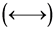Double headed arrow: Double headed arrow is shown between the two canonical structures (resonance structures).

B.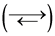Equilibrium symbol: Equilibrium symbol, which favors a forward reaction.

C.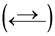Equilibrium symbol: Equilibrium symbol which favors a reverse reaction.

D.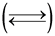Equilibrium symbol: Equilibrium symbol, which favors neither forward nor reverse reaction.

Equilibrium nature: Equilibrium shifts towards less acidic substances that are from lower ${\\rm{P}}{{\\rm{k}}_{\\rm{a}}}$ to higher ${\\rm{P}}{{\\rm{k}}_{\\rm{a}}}$of the substances.

First Step | All Steps | Answer Only

Step-by-step

Step 1 of 2

(A)

The given reaction condition is drawn below:The reaction is drawn below with the best descriptor which represents the reaction.Part AExplanation | Common mistakes | Hint for next step

It is clear that the given reaction represents acid-base reaction where Pyridinium ion acts as acid while pipyridine acts as base. In this reaction, Pyridinium ion gives proton to pipyridine and produces stable pyridine and protonated pipyridine. This reaction equilibrium favor a forward reaction due to the less acidic nature of products than reactants.

Step 2 of 2

(B)

The given reaction condition is drawn below:The reaction is drawn below with the best descriptor which represents the reaction.Part BExplanation | Common mistakes

It is clear that the given reaction represents an acid base reaction where alkyne derivative acts as acid while n-BuLi acts as base. In the reaction, alkyne derivative gives proton to n-BuLi and produces stable butane. This reaction equilibrium favors a forward reaction due to the less acidic nature of products than reactants. Butane has higher${\\rm{P}}{{\\rm{k}}_{\\rm{a}}}$than terminal alkyne derivative.

Part APart BAdd Answer of: (9-8) Select the descriptor (A, B, C, D): that best describes the relationship between the...
More Homework Help Questions Additional questions in this topic.

• Study the following sentence, then select the answer that best describes it

Need Online Homework Help?